# 03.03 Statistics and Plots¶

Plotting functions is all fun but what about the times when we do not actually know the function to plot? When faced with new data it is rare that we actually face a situation with one labeled independent and one labeled dependent variable.

This is where statistics come in, when presented with several dimensions of data we want to be capable of plotting two things:

• several dimensions against each other
• distribution estimates of the dimensions

We have accumulated some boilerplate code to add to the beginning of the notebook, this will keep growing as we start using more tools.

In :
import numpy as np
import matplotlib.pyplot as plt
%matplotlib inline


## The Scatter Plot¶

Since we know that matplotlib simply draws straight lines between the points given to it, if we do not draw the lines and just the markers we get a scatter plot.

In :
fig, ax = plt.subplots(figsize=(13, 5))
x = np.linspace(0, 4, 32)
y = np.exp(x)

ax.plot(x, y, '.');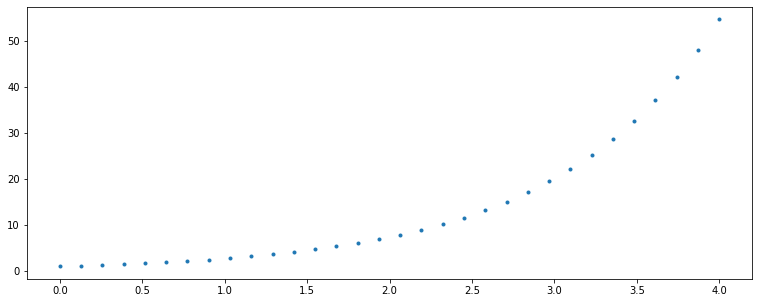That is a plot alright, and it does allow us to plot one dimension against another. In other words, this is a two dimensional plot. But that's about it, we cannot add more dimensions. That seems a fair statement. We are working in two dimensions, therefore we can only compare two dimensions at a time.

Yet, that's an incorrect assumption. We can do more. Apart from the scales on each side of the plot (each axis), one can perceive other attributes inside the plot. Two of those are: the color of a point and the size of each point.

scatter allows us to change the size and color of each point. Let's have a quick look at a four dimensional plot.

In :
x = np.array([1., 5., 7., 9., -3.,  6.])
y = np.array([3., 7., 4., 1.,  5., -1.])
c = np.array([.2, .1, .9, 1.,  .2,  .3])
s = 1024 * c

fig, ax = plt.subplots(figsize=(12, 6))
paths = ax.scatter(x, y, c=c, s=s, alpha=0.5, cmap='viridis')
fig.colorbar(paths);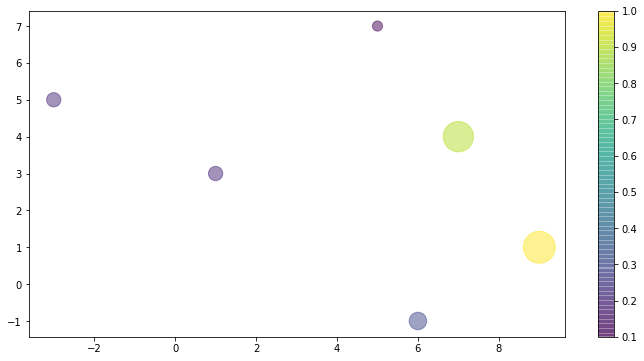More than two dimensions can be used, plt.scatter allows to define the transparency (alpha), and marker of each point. That said, plots with more than four dimension properties start to become difficult to distinguish, (is that point smaller or is it just a smaller marker type?) and are rarely used.

### Note on Colormaps¶

Above we used a color map called viridis (the default in matplotlib), it is a color map which preserves luminosity across its entire color range. The human eye is very good at spotting patters that are not actually there, and more luminous colors may appear bigger which is not desirable most of the time.

On the other hand, if we have a known continuous distribution color maps ranging between two colors only are a better representation. Choosing a good color map for a graph is a difficult task and a big discussion in the visualization field. matplotlib documentation provide some discussion together with its reference for colormaps.plt-iris.svg

## Iris Data¶

A graph without showing actual, meaningful, data does not provide much information. Let's jump a little ahead and download the Iris dataset from Scikit-Learn. This dataset is a collection of four features of Iris flowers, and is often used as an example of classifying Iris species from these features. The dataset itself has a thorough description.

In :
from sklearn.datasets import load_iris
print(iris['DESCR'])

.. _iris_dataset:

Iris plants dataset
--------------------

**Data Set Characteristics:**

:Number of Instances: 150 (50 in each of three classes)
:Number of Attributes: 4 numeric, predictive attributes and the class
:Attribute Information:
- sepal length in cm
- sepal width in cm
- petal length in cm
- petal width in cm
- class:
- Iris-Setosa
- Iris-Versicolour
- Iris-Virginica

:Summary Statistics:

============== ==== ==== ======= ===== ====================
Min  Max   Mean    SD   Class Correlation
============== ==== ==== ======= ===== ====================
sepal length:   4.3  7.9   5.84   0.83    0.7826
sepal width:    2.0  4.4   3.05   0.43   -0.4194
petal length:   1.0  6.9   3.76   1.76    0.9490  (high!)
petal width:    0.1  2.5   1.20   0.76    0.9565  (high!)
============== ==== ==== ======= ===== ====================

:Missing Attribute Values: None
:Class Distribution: 33.3% for each of 3 classes.
:Creator: R.A. Fisher
:Donor: Michael Marshall (MARSHALL%PLU@io.arc.nasa.gov)
:Date: July, 1988

The famous Iris database, first used by Sir R.A. Fisher. The dataset is taken
from Fisher's paper. Note that it's the same as in R, but not as in the UCI
Machine Learning Repository, which has two wrong data points.

This is perhaps the best known database to be found in the
pattern recognition literature.  Fisher's paper is a classic in the field and
is referenced frequently to this day.  (See Duda & Hart, for example.)  The
data set contains 3 classes of 50 instances each, where each class refers to a
type of iris plant.  One class is linearly separable from the other 2; the
latter are NOT linearly separable from each other.

.. topic:: References

- Fisher, R.A. "The use of multiple measurements in taxonomic problems"
Annual Eugenics, 7, Part II, 179-188 (1936); also in "Contributions to
Mathematical Statistics" (John Wiley, NY, 1950).
- Duda, R.O., & Hart, P.E. (1973) Pattern Classification and Scene Analysis.
(Q327.D83) John Wiley & Sons.  ISBN 0-471-22361-1.  See page 218.
- Dasarathy, B.V. (1980) "Nosing Around the Neighborhood: A New System
Structure and Classification Rule for Recognition in Partially Exposed
Environments".  IEEE Transactions on Pattern Analysis and Machine
Intelligence, Vol. PAMI-2, No. 1, 67-71.
- Gates, G.W. (1972) "The Reduced Nearest Neighbor Rule".  IEEE Transactions
on Information Theory, May 1972, 431-433.
- See also: 1988 MLC Proceedings, 54-64.  Cheeseman et al"s AUTOCLASS II
conceptual clustering system finds 3 classes in the data.
- Many, many more ...


We will plot three of these features of the Iris flowers against the actual species. As for the color map we will use plasma, a different luminosity preserving map.

In :
fig, ax = plt.subplots(figsize=(16, 9))
ax.scatter(iris.data[:, 0], iris.data[:, 1],
alpha=0.5, s=256*iris.data[:, 3],
c=iris.target, cmap='plasma',
label='Size depicts {0}'.format(iris.feature_names))
ax.set_xlabel(iris.feature_names)
ax.set_ylabel(iris.feature_names);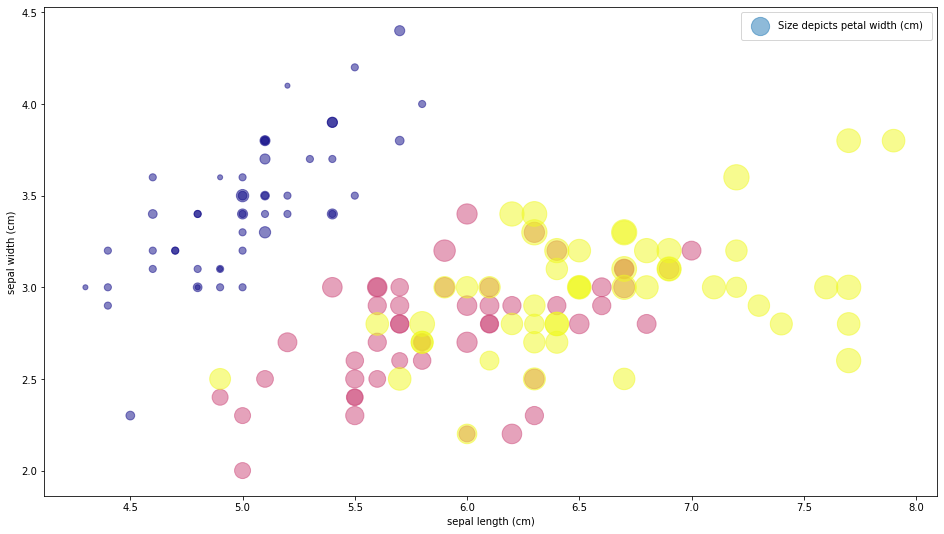We just plotted four different dimensions of the data in a two dimensional plot. The location on the plot of each point corresponds to the sepal length and width, the size of the point is related to the petal width, and the color is related to the particular species of flower.

## The Histogram¶

Nothing better to get a feel for the data than figuring out its distribution. And the quick-and-dirty tool for estimating the distribution is a histogram. hist has lots of customization options, let's see some.

In :
fig, ax = plt.subplots(figsize=(12, 6))
x = np.random.randn(1024)
ax.hist(x, bins=64, alpha=0.5, color='crimson', edgecolor='navy');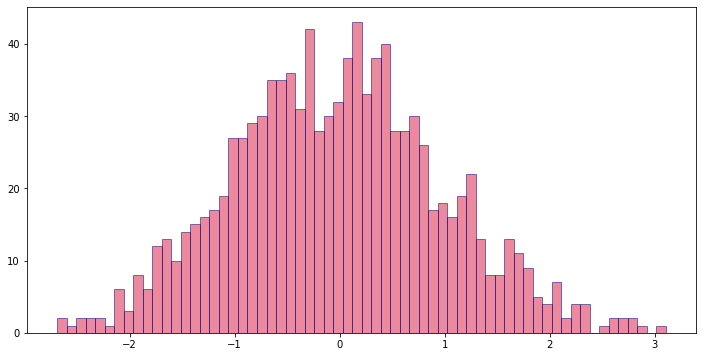Note that if you are after a histogram without actually plotting it, NumPy has its own histogram function.

In :
x = np.random.randn(1024)
hist, bin_edges = np.histogram(x, bins=16)
hist, bin_edges

Out:
(array([  3,   8,  21,  58,  58,  95, 114, 139, 142, 128, 107,  62,  45,
32,   8,   4]),
array([-2.82658784, -2.47230904, -2.11803023, -1.76375143, -1.40947263,
-1.05519383, -0.70091503, -0.34663623,  0.00764257,  0.36192137,
0.71620017,  1.07047897,  1.42475777,  1.77903658,  2.13331538,
2.48759418,  2.84187298]))

### Compare Distributions¶

If we want to compare the distributions against each other, we can normalize the histograms (make the area below the histogram equal a unit) with density=True, and use histtype='stepfilled' to remove the vertical bars. Then we add some transparency to the histograms (alpha=) and we can plot several histograms together.

In :
tall = np.random.normal(0, 0.5, 1024)
neg = np.random.normal(-3, 1, 2048)
fat = np.random.normal(-1, 2, 1024)
kwargs = dict(histtype='stepfilled', alpha=0.5, density=True, bins=64)

fig, ax = plt.subplots(figsize=(13, 6))
ax.hist(tall, **kwargs)
ax.hist(neg, **kwargs)
ax.hist(fat, **kwargs);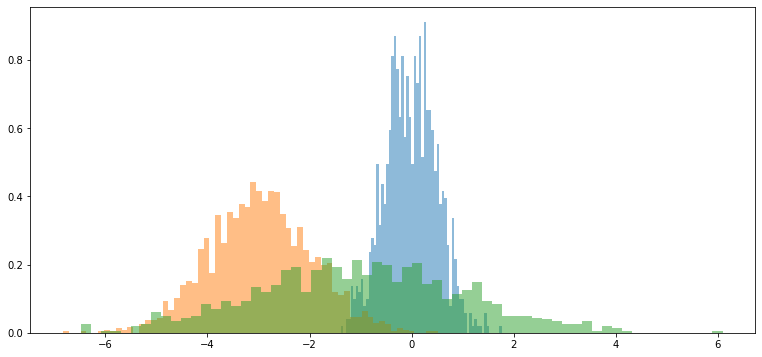## Filling areas¶

Where we will use matplotlib most will be when figuring out whether a model we have built works or does not work. Let's again jump ahead and walk through an sklearn example that requires the use of plt.fill_between.

We will look at a Gaussian Process Regressor model, which is a non-parametric model that can provide us with an estimate of how well it fits the data at each point. In other words, we can know the likeness where unseen data points may be. The Gaussian Process bears similarity to the Random Walk, where we build several paths walking through the space. The difference is that in a random walk all paths start from zero in a gaussian process the starting point of the path is also random. The algorithm then takes in the data provided. All paths that do not pass close enough to the data are then thrown away, in most cases the vast majority of the generated paths is thrown away at this stage. The solution to the regression is the mean of all paths that remain.

We are using code from sklearn, do not worry about this code yet. The common pattern for sklearn models is to import a class which receives parameters when instantiated, which builds the model object. The model object then is trained with the fit method, and performs predictions with the predict method. Later we will go in much more depth about sklearn's models.

Our final objective here is to fill some areas. We will fill in a $95\%$ confidence interval based on the error between the real and the regressed function. For now we assume a gaussian distribution of error and in such a distribution $2\sigma$, i.e. two times the standard deviation, is about a $95\%$ confidence region. Eventually we will look at why this confidence interval is $2\sigma$.

In :
from sklearn.gaussian_process import GaussianProcessRegressor

model = lambda x: x * np.sin(x)
X = np.array([1, 3, 5, 6, 8])
y = model(X)
xfit = np.linspace(0, 10, 1024)

gpr = GaussianProcessRegressor(alpha=1e-3)
gpr.fit(X[:, np.newaxis], y)
yfit = gpr.predict(xfit[:, np.newaxis])
se = ((model(xfit) - yfit)**2)
dyfit = 2 * np.sqrt(se)
me = se.mean()

fig, ax = plt.subplots(figsize=(13, 9))
ax.plot(X, y, 'or', label='observations')
ax.plot(xfit, yfit, '-', color='steelblue', label='prediction')
ax.plot(xfit, model(xfit), '-', color='red', alpha=0.5, label='$f(x) = x \cdot sin(x)$')
ax.fill_between(xfit, yfit - dyfit, yfit + dyfit, color='gray', alpha=0.2, label='95% confidence')
ax.legend(loc='upper left')

me

Out:
1.605808860869753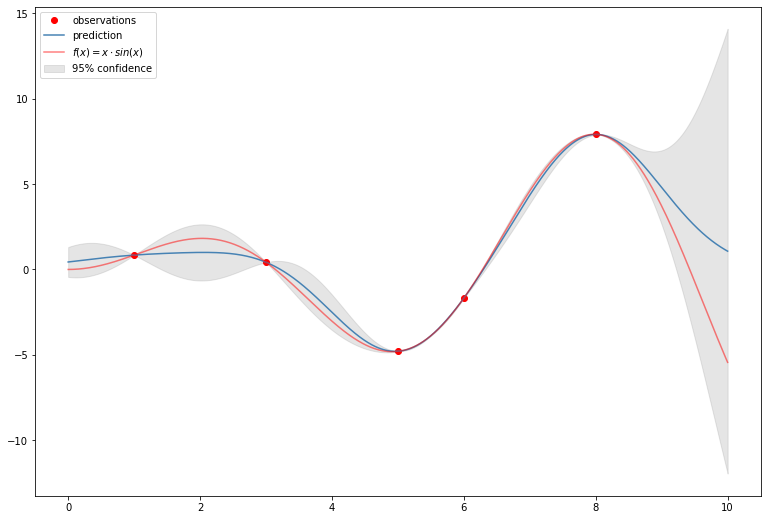plt.fill_between receives two mandatory and at least one optional argument. The first arguments produce the function to fill the area to, if a third argument is not given everything from $y=0$ will be filled. The third argument, if given, is the function (the y values) to which we fill to (instead of zero).

The remaining arguments are to style the lines produced, this is another common pattern in matplotlib.